Science, Maths & Technology

### Become an OU student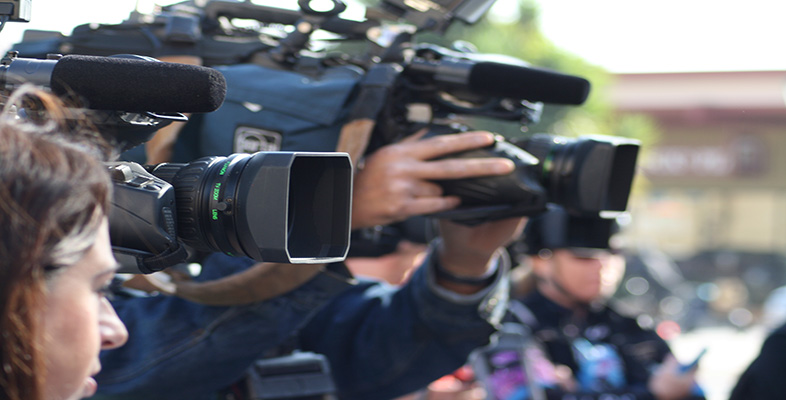IT: Information

Start this free course now. Just create an account and sign in. Enrol and complete the course for a free statement of participation or digital badge if available.

# 4.2.5 Focusing

Focusing is done by adjusting the size of the gap between the lens and the light sensor. To get distant objects in focus, the gap needs to be smaller than that required for close objects (see Figure 7 below).

In theory the exact gap size is determined by the exact distance to the object being filmed. In practice, one gap size will be adequate for a range of object distances, this range being called the 'depth of field'.

Focusing in a camcorder is invariably done with an electric motor moving a lens, and there will be a facility to focus automatically (autofocus). Auto focusing is either passive or active.

Passive autofocusing works by a computer embedded in the camera examining the image (from the light sensor) to determine whether it is in focus or not.

## Activity 12

How do you decide whether an image is in focus? What do you think the camera's computer can look for to determine whether the image is in focus?

### Discussion

You can tell whether an image is in focus by seeing how 'sharp' it is. The camera's computer looks for sharp edges – sudden changes in colour or brightness. These abrupt changes will be present only if the image is in focus.

Under control of the camera's computer, the motor will move the lens in and out to find the best focus, identified by the presence of sharp lines in the image.

Active autofocusing works by the camera measuring the distance to the object viewed, and using that to calculate the gap needed between lens and light sensor. It measures the distance by sending out pulses of infrared light towards the object being filmed, and measuring how long it takes the reflected light to get back to the camera. During this time, between sending the pulse and detecting the reflected pulse, the light does a round trip – it travels out and back again. The time the light takes to go one way is therefore half the measured interval. I'll call this time – half the time between sending and receiving the pulse – the transit time.

The speed of light is known, so the transit time can be used to calculate the distance between the camera and the object.

Specifically, the distance from the camera to the object is given by the transit time multiplied by the speed of light.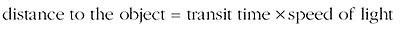This can be written much more concisely using

• d for the distance to the object

• t for the transit time

• c for the speed of light.

Strictly c is the speed of light in free space (a vacuum). In the atmosphere – through air – light travels at almost the same speed as in a vacuum, and we can neglect the difference. In glass, however, light travels substantially slower (about two-thirds the speed) and it is necessary to take account of this fact when considering light in optical fibre.

Then I can write: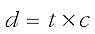When writing equations like this, it is a convention that you can miss out the multiplication symbol. Any numbers or letters written next to each other are taken to be multiplied together, so we can write: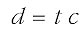Equations relating three quantities in this particular way, where one (in this case d) is given by multiplying the other two (in this case t and c) together, are quite common, and you will be meeting other examples later in this course. For reasons which will become clearer later, it is useful to draw this type of equation in a 'formula triangle', as shown in Figure 8. The quantities that are multiplied together go in the bottom two corners (it doesn't matter which way around), and the thing they calculate goes in the top corner. I am not going to say anything more about the formula triangle for the moment, though it might be a bit mysterious, but it will become clearer later.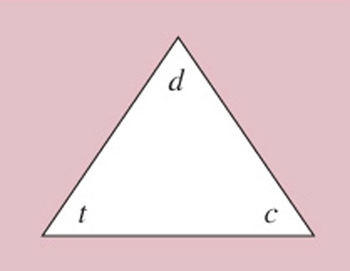Figure 8 A formula triangle

I now want to put in a value for the speed of light, c, so that I will have a formula that allows me to calculate the distance d directly from the transit time t.

The speed of light in metres per second is 3×108. That is to say, light travels 3×108=300,000,000 metres every second. When I am doing calculations related to the focusing of the camera, however, I will find the times I am using will be much smaller than a second and the relevant distances will usually be of the order of a few metres – not three hundred million metres! What I am going to do, therefore, is express the speed of light in terms of how far it travels in one nanosecond (ns), which is one thousand-millionth of a second: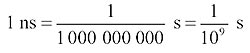## Activity 13

How far will light travel in one nanosecond?

### Discussion

Light travels 300,000,000 metres in one second, so in one nanosecond it travels: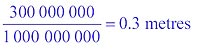So I can express the speed of light as 0.3 m/ns (0.3 metres per nanosecond). But this means that when I write the equation, t represents a time in nanoseconds and d represents a distance in metres. It is important that all the units match. Using a value of c=0.3 m/ns and provided t and d are in nanoseconds and metres respectively, I get: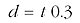It doesn't matter which way round you write a multiplication (4×5 is the same as 5×4) and it is a convention always to put numbers before letters, so this would normally be written: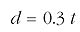For example, if the transit time is 20 ns, the distance in metres is given by: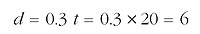So the distance is 6 m.

## Activity 14

If the transit time is 14 ns, how far away is the object?

### Discussion

The distance in metres is given by: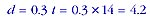So the distance is 4.2 m.

## Algebra and the use of symbols

The use of symbols to represent numerical values, such as 'd ' for distance, 't' for time, 'c' for the speed of light, is the starting point for algebra. If you don't like maths this might be worrying, but I hope that when you get used to it – and simple familiarity goes a long way to demystifying algebra – you will see that at least it provides useful shorthand.

If you know that c is being used to represent the value for the speed of light and t a time duration, then you will automatically read c×t as 'multiply the speed of light by the time duration'. It is helped – when you are used to it – by the fact that the speed of light is nearly always represented by c, and that t refers to time in lots of different contexts. Notice, incidentally, that there is a subtle difference in the use of c compared with d and t, because c is a fixed number, but d and t can change. We say that c is a 'constant' whereas d and t are 'variables'.

Besides the use of symbols being a shorthand, there is much that you can do by 'manipulating' them, but you will only be meeting this at a simple level in this course. There is more about algebra in The Sciences Good Study Guide, Maths Help, Section 9 (Northedge et al., 1997).

Remember that the reason for discussing this calculation was to show that when using active focusing the camcorder can measure the distance to an object by transmitting and detecting an infrared pulse. Active autofocusing therefore involves another output from and input to the camcorder (sending and receiving the infrared pulse). We can show this by adding it to the high-level diagram of a camcorder that was shown in Figure 4, to get that shown in Figure 9.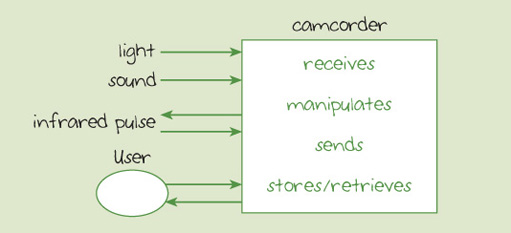Figure 9 Model of camcorder with active autofocusing

At a lower level of abstraction, the components of the active autofocusing are shown in Figure 10, together with other relevant components of the camcorder.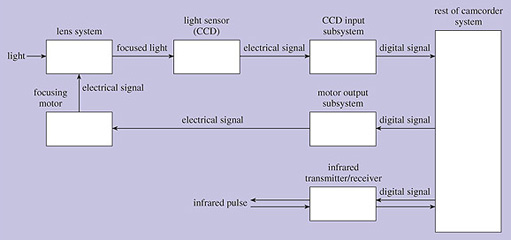Figure 10 Active autofocusing

Figure 10 is similar to Figure 5, but I have removed the detail of the sound subsystem in order to concentrate on the light and focusing subsystems.

## Activity 15

Write down what you think is done by each of the following boxes in Figure 10